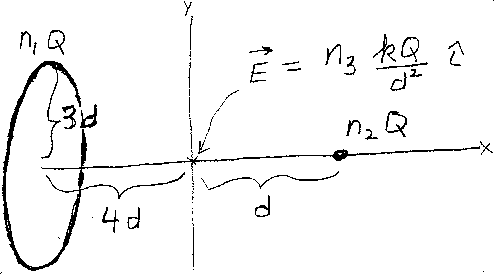Problem A14: In the figure above, there is a charged non-conducting ring of radius 3d, and a small charged object. The ring has a charge of n1Q, and the center of the ring is located on the x-axis at x=-4d. The small "point" object has a charge of n2Q and is located on the x-axis at x=d. What is the electric field at the origin? That is, if the electric field at the origin equals E = n3 kQ/d2 i , then what is n3 ?n1 = n2 = Input n3:

If you are currently in my class, you can record your grade by entering your name and student ID number (without the leading zeros) below and clicking on "record grade".
 First Name = Last Name = ID = Problem: